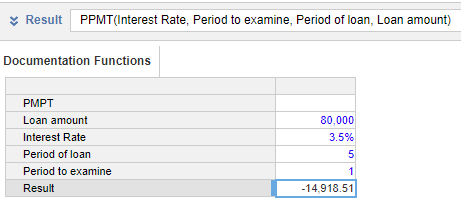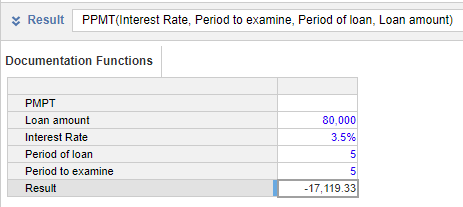1. Calculation functions
2. All Functions
3. Financial functions
4. PPMTThe PPMT function calculates the amount of a payment allocated to the principal part of a loan.

This function assumes equal payment amounts over the period of the loan.

The period passed in any argument in the function must be is consistent. The function takes up to six arguments: the first argument (i) requires the interest rate in the calculation period; the second (c) and third (p) arguments specify the periods used in the calculation. These periods must match, so to calculate the annual principal paid, use the annual interest rate, and the number of periods in years. Bear this in mind when using hard-coded values in the function.

This function is equivalent to the Excel function PPMT.

Output

The function must be used in a line item with the Format: Number.

Syntax

PPMT(i, c, p, v, [f], [mode])
Argument Description Format Available Sources
i interest rate per period Number (percentage) Numeric hard-coded value, line item, or property
c the period to check Number Numeric hard-coded value, line item, or property
p the number of periods over which the loan is repaid Number Numeric hard-coded value, line item, or property
v the current loan balance Number Numeric hard-coded value, line item, or property
f (optional) the residual amount due after the last payment is made. If this argument value is zero or not provided, the function assumes the residual value is zero. Number Numeric hard-coded value, line item, or property
mode (optional) when the payments are made. If the value is not provided, or the value is zero, the function assumes payments are made at the end of each period; a non-zero value means payments are made at the start of each period. Number

Numeric hard-coded value, line item, or property

Example

The formula to calculate the principal paid in the first annual payment is:

PPMT(Interest Rate, Period to examine, Period of loan, Loan amount)

where the line items use this data:

Line item Value
Loan amount 80000
Interest Rate 3.5
Period of loan 6
Period to examine 1The Period of loan is in years, matching the annual Interest Rate. The Period to examine specifies the payment in year one. The argument to specify when the loan payment is made (mode) is not included, so is assumed to be at the end of the year.

By changing the Period to examine to 5, the formula shown below calculates the principal paid in the last year of the same loan.